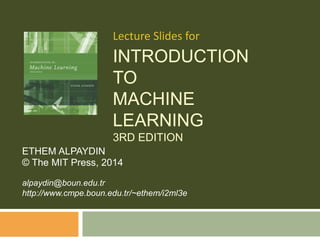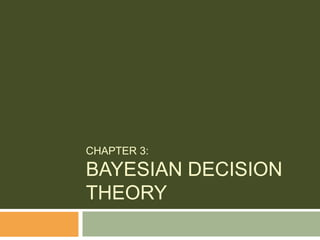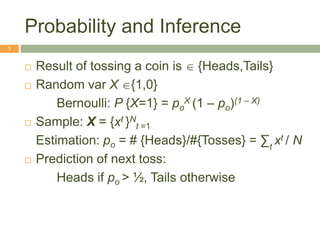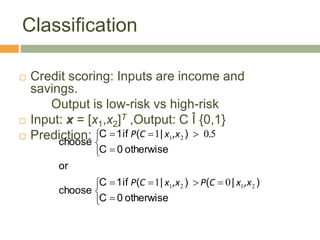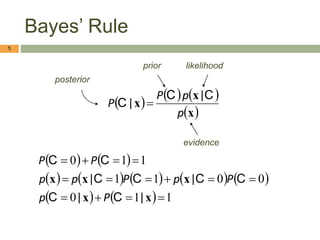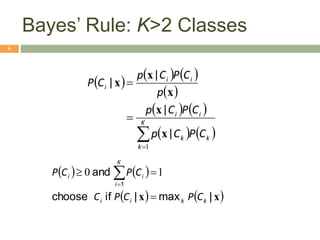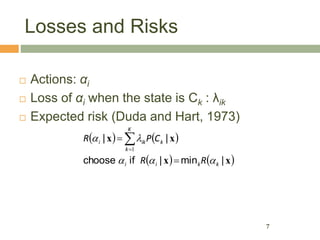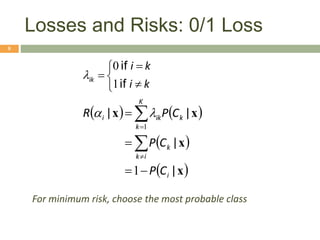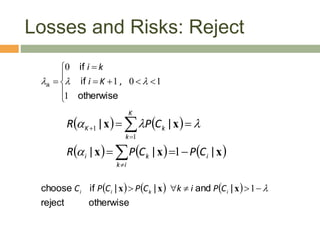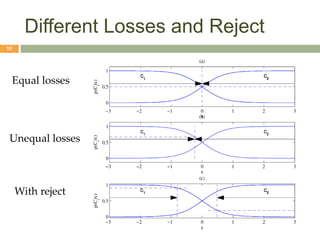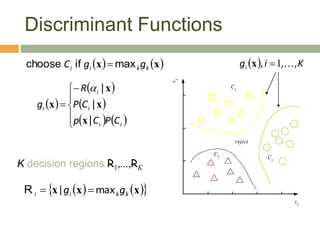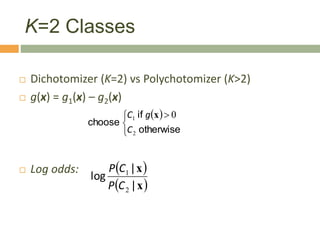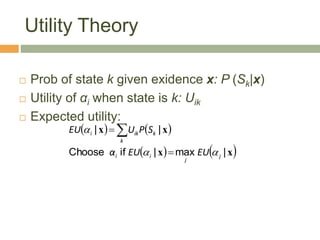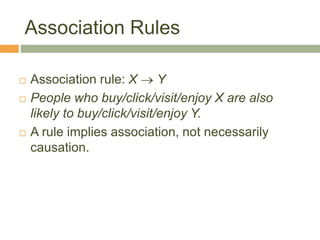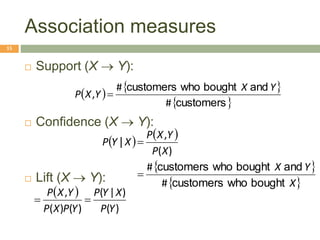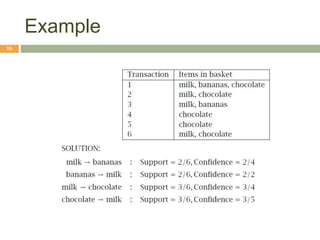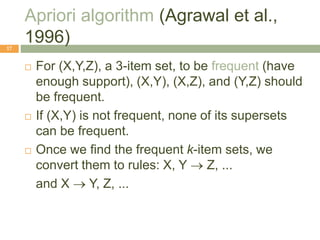1 de 17

### i2ml3e-chap3.pptx

1. INTRODUCTION TO MACHINE LEARNING 3RD EDITION ETHEM ALPAYDIN © The MIT Press, 2014 alpaydin@boun.edu.tr http://www.cmpe.boun.edu.tr/~ethem/i2ml3e Lecture Slides for
2. CHAPTER 3: BAYESIAN DECISION THEORY
3. Probability and Inference 3  Result of tossing a coin is {Heads,Tails}  Random var X {1,0} Bernoulli: P {X=1} = po X (1 ‒ po)(1 ‒ X)  Sample: X = {xt }N t =1 Estimation: po = # {Heads}/#{Tosses} = ∑t xt / N  Prediction of next toss: Heads if po > ½, Tails otherwise
4. Classification  Credit scoring: Inputs are income and savings. Output is low-risk vs high-risk  Input: x = [x1,x2]T ,Output: C Î {0,1}  Prediction:                otherwise 0 ) | ( ) | ( if 1 choose or otherwise 0 ) | ( if 1 choose C C C C ,x x C P ,x x C P . ,x x C P 2 1 2 1 2 1 0 1 5 0 1 4
5. Bayes’ Rule         x x x p p P P C C C | |                    1 1 0 0 0 1 1 1 1 0               x x x x x | | | | C C C C C C C C P p P p P p p P P 5 posterior likelihood prior evidence
6. Bayes’ Rule: K>2 Classes                     K k k k i i i i i C P C p C P C p p C P C p C P 1 | | | | x x x x x         x x | max | if choose and 1 k k i i K i i i C P C P C C P C P      1 0 6
7. Losses and Risks  Actions: αi  Loss of αi when the state is Ck : λik  Expected risk (Duda and Hart, 1973)         x x x x | min | if choose | | k k i i k K k ik i R R C P R         1 7
8. Losses and Risks: 0/1 Loss       k i k i ik if if 1 0          x x x x | | | | i i k k K k k ik i C P C P C P R         1 1   8 For minimum risk, choose the most probable class
9. Losses and Risks: Reject 1 0 1 1 0               otherwise if if , K i k i ik           x x x x x | | | | | i i k k i K k k K C P C P R C P R           1 1 1           otherwise reject | and | | if choose       1 x x x i k i i C P i k C P C P C 9
10. Different Losses and Reject 10 Equal losses Unequal losses With reject
11. Discriminant Functions   K i gi , , ,  1  x     x x k k i i g g C max if choose        x x x k k i i g g max |   R                 i i i i i C P C p C P R g | | | x x x x  11 K decision regions R1,...,RK
12. K=2 Classes  Dichotomizer (K=2) vs Polychotomizer (K>2)  g(x) = g1(x) – g2(x)  Log odds:       otherwise if choose 2 1 0 C g C x     x x | | log 2 1 C P C P 12
13. Utility Theory  Prob of state k given exidence x: P (Sk|x)  Utility of αi when state is k: Uik  Expected utility:         x x x x | max | if Choose | | j j i i k k ik i EU EU α S P U EU       13
14. Association Rules  Association rule: X  Y  People who buy/click/visit/enjoy X are also likely to buy/click/visit/enjoy Y.  A rule implies association, not necessarily causation. 14
15. Association measures 15  Support (X  Y):  Confidence (X  Y):  Lift (X  Y):       customers and bought who customers # # , Y X Y X P          X Y X X P Y X P X Y P bought who customers and bought who customers | # # ) ( ,     ) ( ) | ( ) ( ) ( , Y P X Y P Y P X P Y X P  
16. Example 16
17. Apriori algorithm (Agrawal et al., 1996) 17  For (X,Y,Z), a 3-item set, to be frequent (have enough support), (X,Y), (X,Z), and (Y,Z) should be frequent.  If (X,Y) is not frequent, none of its supersets can be frequent.  Once we find the frequent k-item sets, we convert them to rules: X, Y  Z, ... and X  Y, Z, ...